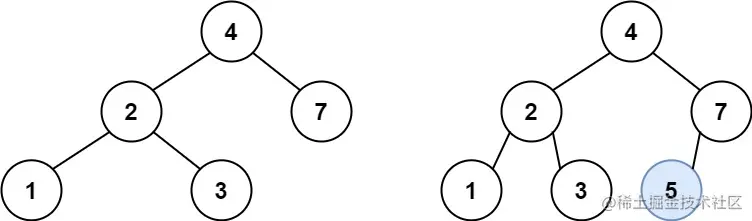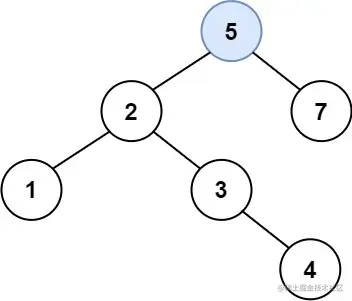# leetcode 701. Insert into a Binary Search Tree （python）

2022-01-30 03:13:34

This article has participated in 「 Digging force Star Program 」, Win a creative gift bag , Challenge creation incentive fund

### describe

You are given the root node of a binary search tree (BST) and a value to insert into the tree. Return the root node of the BST after the insertion. It is guaranteed that the new value does not exist in the original BST.

Notice that there may exist multiple valid ways for the insertion, as long as the tree remains a BST after insertion. You can return any of them.

Example 1:``````Input: root = [4,2,7,1,3], val = 5
Output: [4,2,7,1,3,5]
Explanation: Another accepted tree is:
Copy code ``````Example 2:

``````Input: root = [40,20,60,10,30,50,70], val = 25
Output: [40,20,60,10,30,50,70,null,null,25]
Copy code ``````

Example 3:

``````Input: root = [4,2,7,1,3,null,null,null,null,null,null], val = 5
Output: [4,2,7,1,3,5]
Copy code ``````

Note:

``````The number of nodes in the tree will be in the range [0, 10^4].
-10^8 <= Node.val <= 10^8
All the values Node.val are unique.
-10^8 <= val <= 10^8
It's guaranteed that val does not exist in the original BST.
Copy code ``````

### analysis

According to the meaning , Is to give the root node of a binary tree root , Then give a value val , The title requires us to val The node of value is inserted into the binary tree , And return to the root node , The title guarantees val It doesn't exist in root Value , And there may be many kinds of binary trees , Just return any one of them .

Or the old way to use recursion to solve problems , Here we only need to know the basic properties of binary tree , That is, the value of the left subtree is smaller than that of the root node , The value of the right subtree is larger than that of the root node . Then use recursion ：

• If the root node root It's empty , Then use val Initialize a node and return
• If the root node root The value is less than val , explain val belong root The right subtree , So recursion is right root Recursive node insertion for the right subtree of
• If the root node root The value is greater than val , explain val belong root The left subtree , So recursion is right root Recursive node insertion in the left subtree of
• Finally, return the current root node root

``````class TreeNode(object):
def __init__(self, val=0, left=None, right=None):
self.val = val
self.left = left
self.right = right
class Solution(object):
def insertIntoBST(self, root, val):
"""
:type root: TreeNode
:type val: int
:rtype: TreeNode
"""
if not root:
node = TreeNode(val)
return node
if root.val < val:
root.right = self.insertIntoBST(root.right, val)
else:
root.left = self.insertIntoBST(root.left, val)
return root

Copy code ``````

### Running results

``````Runtime: 166 ms, faster than 23.65% of Python online submissions for Insert into a Binary Search Tree.
Memory Usage: 17.6 MB, less than 41.28% of Python online submissions for Insert into a Binary Search Tree.
Copy code ``````

### analysis

In fact, I use a clumsy idea , You can do it in several steps ：

• Initialize a L The empty list is used to store all node values
• Definition getListFromBST The function uses recursion to traverse the values of all nodes , Save all L in
• take val Also joined L And then to L Sort
• Defined function sortedListToBST Use recursion to build a binary tree for the list that has been ascending , This operation is the same as this problem leetcode 108. Convert Sorted Array to Binary Search Tree（python）

Obviously, this method is a lot cumbersome , It's not as concise as the previous method .

``````class TreeNode(object):
def __init__(self, val=0, left=None, right=None):
self.val = val
self.left = left
self.right = right
class Solution(object):
def __init__(self):
self.L = []

def getListFromBST(self, root):
if not root:return
self.getListFromBST(root.left)
self.L.append(root.val)
self.getListFromBST(root.right)

def sortedListToBST(self, nums):
if not nums: return
MID = len(nums) // 2
root = TreeNode(nums[MID])
root.left = self.sortedListToBST(nums[:MID])
root.right = self.sortedListToBST(nums[MID+1:])
return root

def insertIntoBST(self, root, val):
"""
:type root: TreeNode
:type val: int
:rtype: TreeNode
"""
self.getListFromBST(root)
self.L.append(val)
self.L.sort()
return self.sortedListToBST(self.L)
Copy code ``````

### Running results

``````Runtime: 389 ms, faster than 5.01% of Python online submissions for Insert into a Binary Search Tree.
Memory Usage: 19.4 MB, less than 16.83% of Python online submissions for Insert into a Binary Search Tree.
Copy code ``````